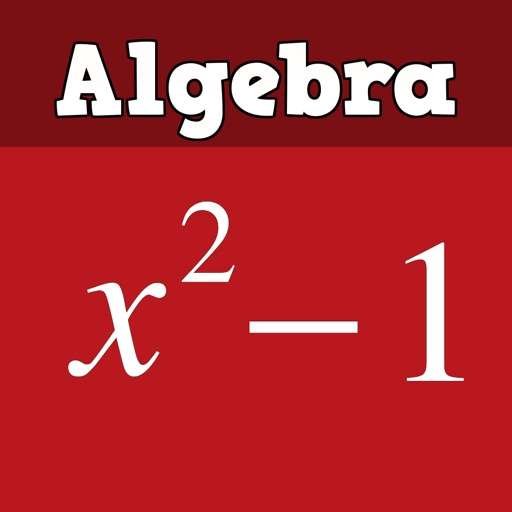## Algebra isn an app that teaches you how to do algebra with worked, step-by-step examples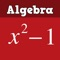# Algebra - Learn math by Example with Problems and Solutions in Self-Teaching Algebra Study Guide

by Flamethrower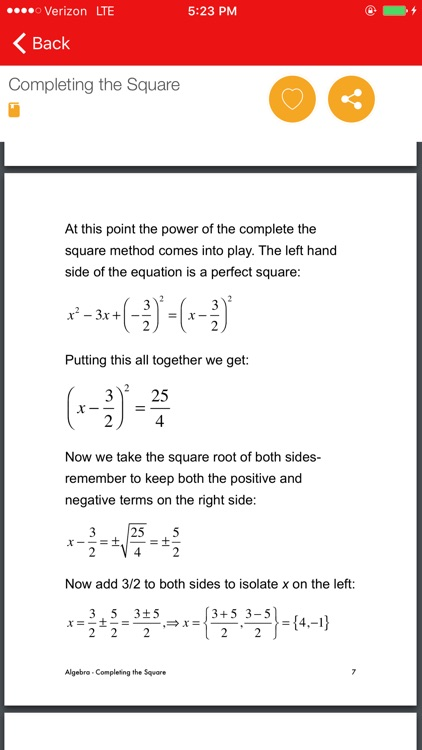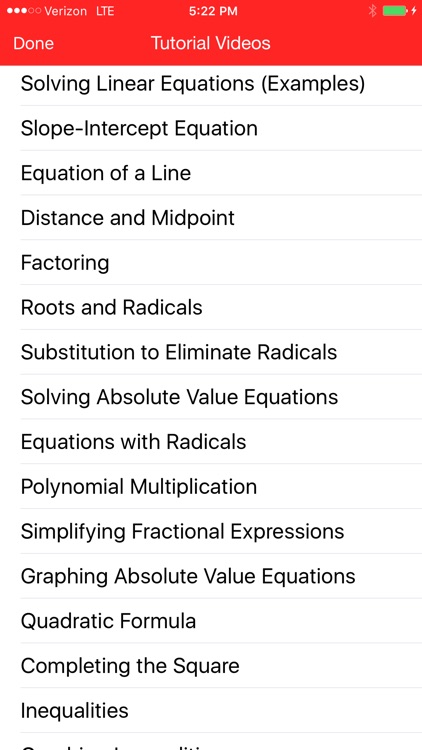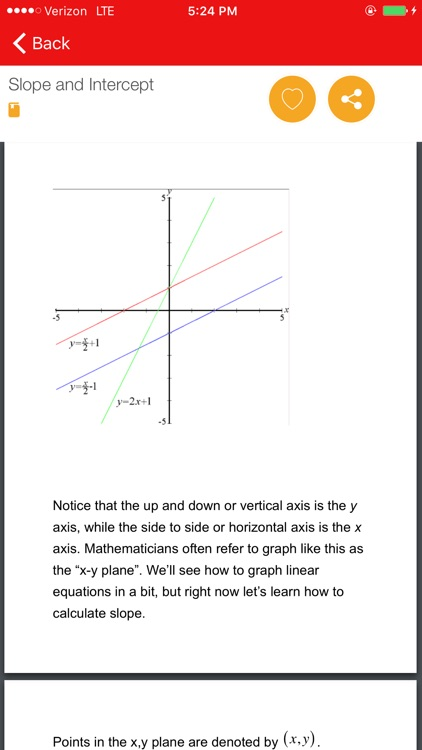Algebra isn an app that teaches you how to do algebra with worked, step-by-step examples. If algebra leaves you confused, Algebra app is here to help with hundreds of worked examples that show step-by-step solutions. This Algebra app is practical - it will help you learn algebra by seeing how an expert solves problems. Includes both books to read at your own pace and video tutorials.### App Details

Version
1.0
Rating
(4)
Size
31Mb
Genre
Education Reference
Last updated
September 8, 2016
Release date
August 19, 2016

### App Screenshots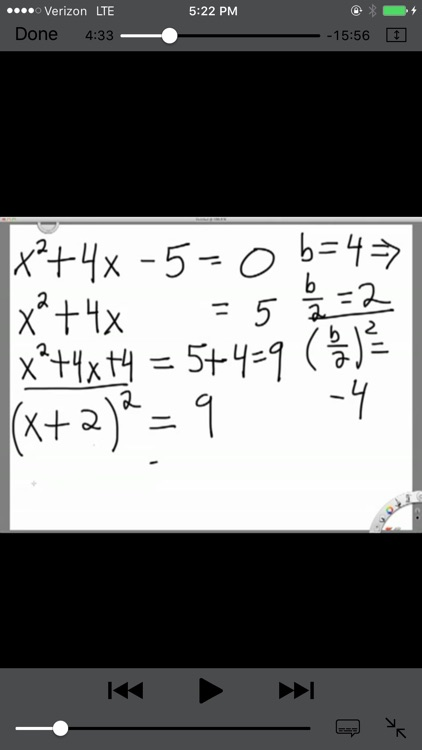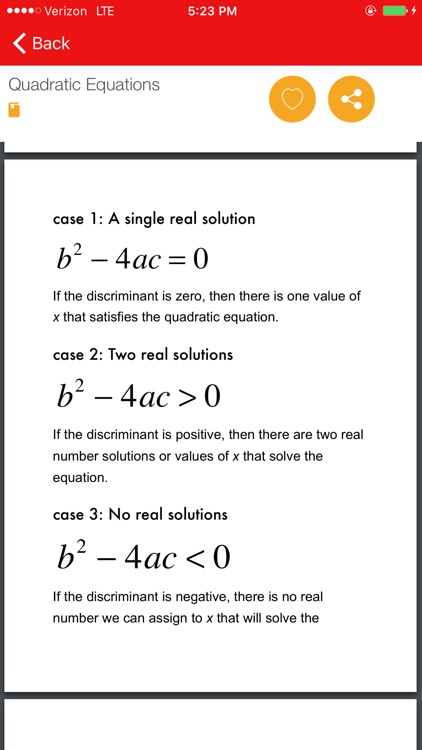### App Store Description

Algebra isn an app that teaches you how to do algebra with worked, step-by-step examples. If algebra leaves you confused, Algebra app is here to help with hundreds of worked examples that show step-by-step solutions. This Algebra app is practical - it will help you learn algebra by seeing how an expert solves problems. Includes both books to read at your own pace and video tutorials.

- Review of numbers and exponents
- Solving for variables
- Factoring
- Rational functions
- Simplifying
- Doing word problems
- Completing the Square
- Solving cubics

Video Tutorials Include:
-Exponents and Absolute Values
- Solving for unknown variables
- Adding, Subtracting, Multiplying and Dividing Polynomials
- Slope-Intercept Equation
- Equation of a Line
- Distance and Midpoint
- Solving Absolute value equations, graphing absolute values
- Simplifying Fractional Expressions
- Completing the Square
- Inequalities
- Interest rate and percentage problems
- Word Problems
- Logarithms
- Solving Simultaneous Equations

FEATURES:
- Easy to understand and learn algebra at your own pace
- Good for all levels of algebra, from algebra I to college algebra
- Great for students or for review and self-study
- Randomly selects a different lesson each day you open the app, or view lessons in order